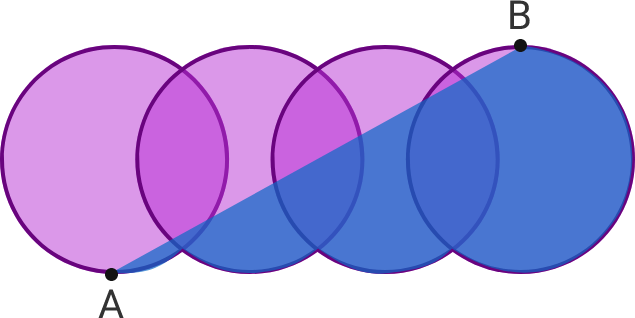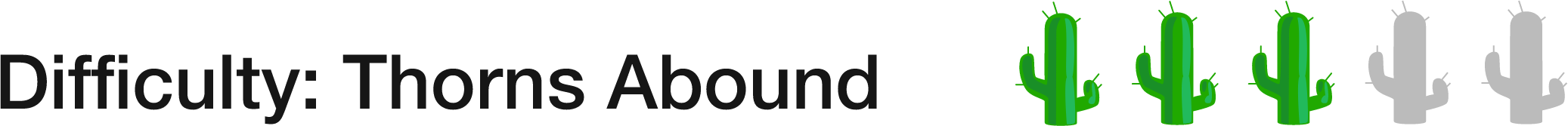# 55 of 100: Rings

Geometry Level 1Facts:

• These four congruent circles are aligned horizontally.
• The area of the entire blue shaded portion of the figure is 22.
• The area of the overlap between each pair of congruent circles is 4.
• A is the lowest point on the left-most circle and B is the highest point on the right-most circle.

What is the area of one of the circles?Start by figuring out the area of the four-overlapping-circle figure.

×